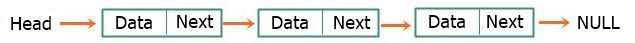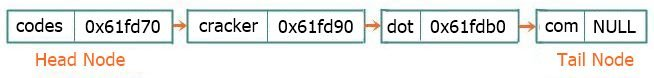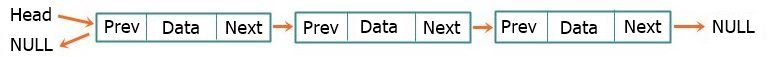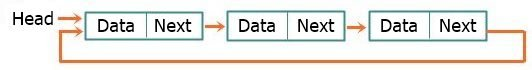# Linked List in Data Structure

The term "list" refers to a linear collection of data. One form of a linear list is an array. As you know, the linear relationship between the data elements of an array is reflected by the physical relationship of the data in the memory (i.e., sequential allocation) and not by any information contained in the data elements themselves. A list, on the other hand, can be stored as having data elements pointing to the next in the sequence (i.e., linked allocation), resulting in a linked list.

Linked lists are a sequence of nodes that each contain a pointer to the next node in the sequence. The structure of a node consists of two parts:

• The first part, data, contains information about the element.
• The next node's address is stored in the second part, which is referred to as the link or next pointer.

The simplest one of these data structures, i.e., an array, can be used only when its number of elements and element sizes are predetermined, since memory is reserved before processing. For this reason, arrays are called dense lists or static data structures. Now consider a situation where the exact number of elements is not known. In such a case, estimates might go wrong. Also, during processing, either more memory is required or extra free spaces lie in the array. Another problem associated with arrays is the complexity involved in the insertions and deletions of elements.

## Memory Allocation (Dynamic vs. Static)

Memory is allocated to each data element stored in memory.This process of allocating memory is called memory allocation. The memory (main memory) can be allocated in two ways:

• dynamically: The number of elements to be stored must be known in advance when using Static Memory Allocation techniques because they reserve a fixed amount of memory before processing begins. Static memory allocation refers to this specific method of allocating RAM. To store arrays, static memory allocation is used.
• statically: Dynamic memory allocation is a technique that allows for memory to be allocated on-the-fly within an operating system or an application. Dynamic memory allocation describes this method of allocating RAM. When memory is no longer needed, it can be released more easily thanks to dynamic allocation. Memory allocation in data structures like linked lists and trees is performed in this way.

The types of linked lists are as follows:

A linear data structure that can only be traversed in one direction is called a "singly linked list." To help you visualize the relationship between the list's nodes and their contents, I drew a little diagram. Let me show you the picture, and then I'll explain it.The above singly linked list contains three nodes, each of which has two parts: one for storing data and the other for storing the address of the next node. Each node in the singly linked list is thus connected in this manner.

The first node is known as the "head" node, and the last node is known as the "tail" node. The "head" node contains the data as well as the address of the next node, whereas the "tail" node contains the data as well as the address "NULL" to end the list. Take a look at the following example:In the figure above, "codes," "cracker," "dot," and "com" are the data of four nodes, and "0x61fd70" is the address of the node (the first or head node) with "cracker" as its data; that is, the node next to this address. Similarly, "0x61fd90" is the address of the third node, the node with "dot" as its data, and so on. Finally, always use "NULL" as the address of the last or tail node to end the list.

When "NULL" is used as the address or second part of the last or tail node, it indicates that the node is pointing to NULL (no address) and causes the list to terminate.

Because each node in a doubly linked list has the three parts or sections listed below, they can be traversed in both directions, front and back.

• The first part contains the previous node's address.
• The data is contained in the second section.
• The third part contains the next node's address.

The snapshot below depicts how nodes in a doubly linked list data structure are organized.Unlike a "singly linked list," the address of the first node is contained in the last node of a circularly linked list. Consider the following image as an example of a circular linked list:Data Structure Quiz

« CodesCracker Next Tutorial »